# Sets Operation in Python Programming

in STEMGeeks5 months ago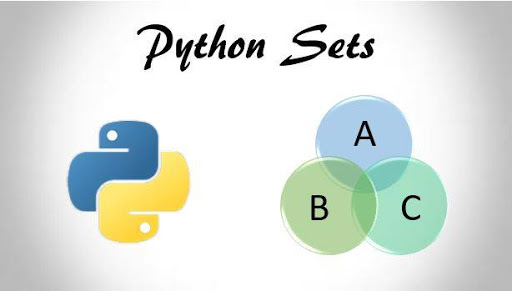Image Source

I have discussed about the basic concepts of sets in python along with examples in one of my previous post which can be found here. In this post, I am gonna write about various operations that we can do with sets like we used to do in mathematics in our school such as union, difference, intersection and so on. You can use any IDE of your choice. Just create a new python file and you can start along with me😀.

In order to remove any items from the sets, you can use 3 built-in functions as we did in list. Lets see how `remove()` function works for sets in below example:

``````my_set = {1, 2, 3, 4, 5}
print("Original set is", my_set)
my_set.remove(2)
print("\nSet after deleting is", my_set)
``````

The `remove()` function takes in the value as an argument that you want to remove. In the above example we want to remove 2 from our set, so we write `remove(2)` and execute it. It will show the following output: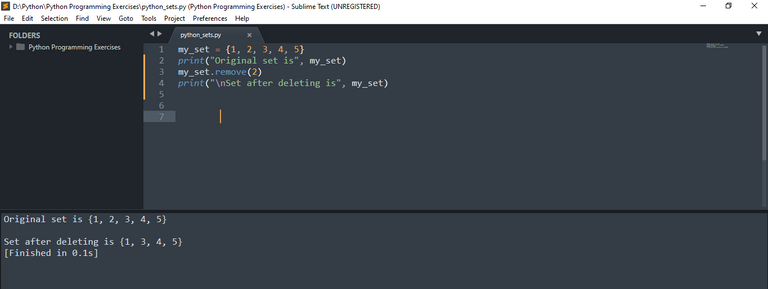There's another method similar to `remove()` in python which is `discard()`. Both function serves the same purpose i.e. it deletes item from the sets that user has desired. The difference is that `remove()` raises an exception error if the element is not found while `discard()` doesn't raise any exception. For example in the above code, we don't have element named as 6 in the set. Lets try removing that one and see what our output looks like: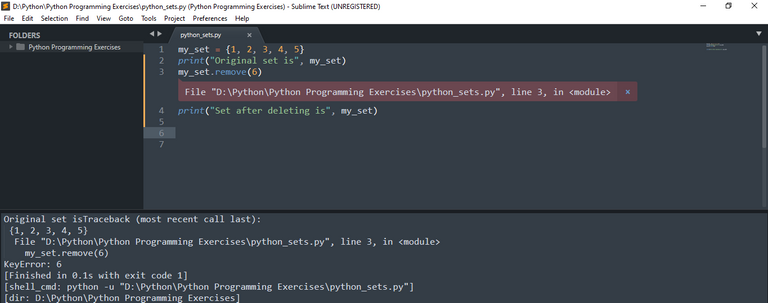You can see it causes an error in the code. Lets try to replace `my_set.remove(6)` with `my_set.discard(6)`.

``````my_set = {1, 2, 3, 4, 5}
print("Original set is", my_set)
print("Set after deleting is", my_set)
``````

Now you won't see any error if the element is not found.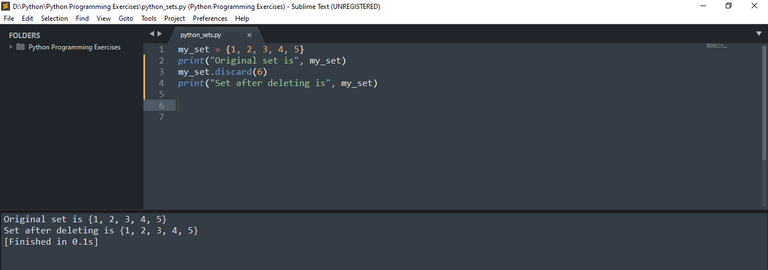Remember, this function will also delete items if the element is found like remove as I just mentioned above. There is last one built-in function called `pop()` that is used to delete random element from the set. Lets see its example as below:

``````my_set = {31, 25, 73, 49, 5, 36, 77}
print("Original set is", my_set)
removed_element = my_set.pop()
print("\nThe removed element is:", removed_element)
print("\nSet after deleting is", my_set)
``````

If you run this program, it will show following output. Remember sets has no particular order so you can see element being printed in random order and pop method removing elements randomly.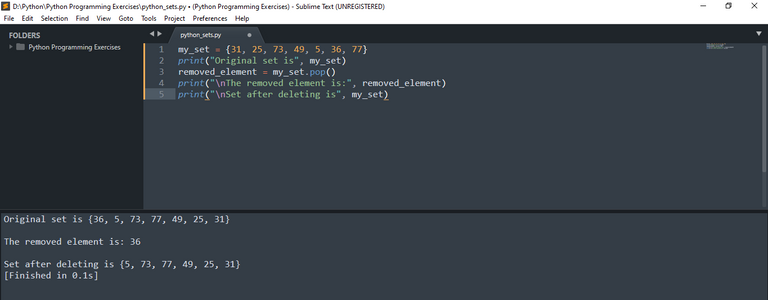Now lets see some major sets operations. First we will see program for intersection of sets. It can be done with the help of `intersection()` function as below:

``````set1 = {1, 2, 3, 4, 5, 6, 7, 8, 9, 10}
set2 = {1, 5, 7, 9}
set3 = {2, 4, 7, 9}

set4 = set1.intersection(set2)
print ("The intersection of set1 and set2 is:", set4)

set5 = set1.intersection(set2, set3)
print("\nThe intersection of set1, set2 and set3 is:", set5)
``````

Running the above code will show you following output. I don't think I need to explain anything here as it is very simple to understand and intersection function can accepts any number of arguments as long as they are defined in the program with correct syntax.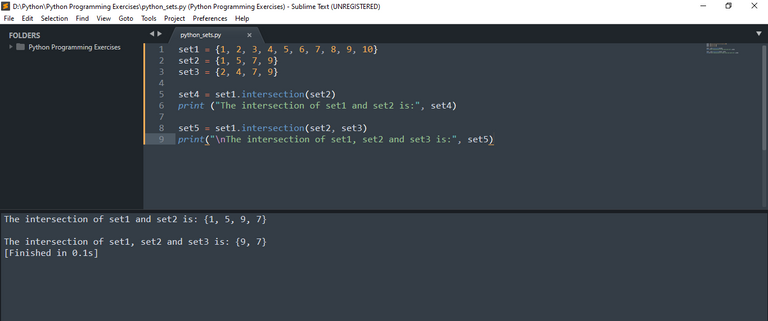Now lets see program for finding union of two sets using `union()` function.

``````set1 = {1, 3, 5, 7, 9}
set2 = {2, 4, 6, 8, 10}

set3 = set1.union(set2)
print ("The union of set1 and set2 is:", set3)
``````

The output of this code is: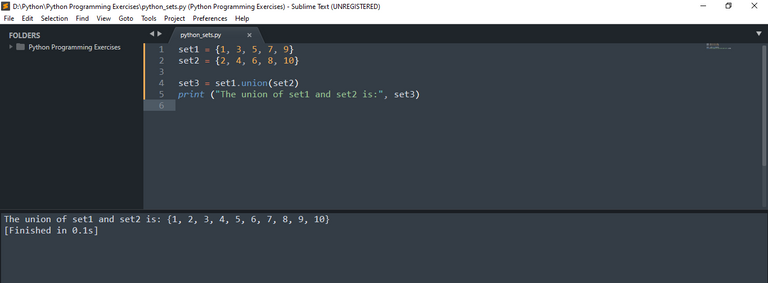Now, lets see how you can achieve difference of two sets using `difference()` function.

``````set1 = {1, 2, 3, 4, 5, 6, 7, 8, 9, 10}
set2 = {2, 4, 6, 8, 10}

set3 = set1.difference(set2)
print ("The difference of set2 from set1 is:", set3)
``````

This will print the following output: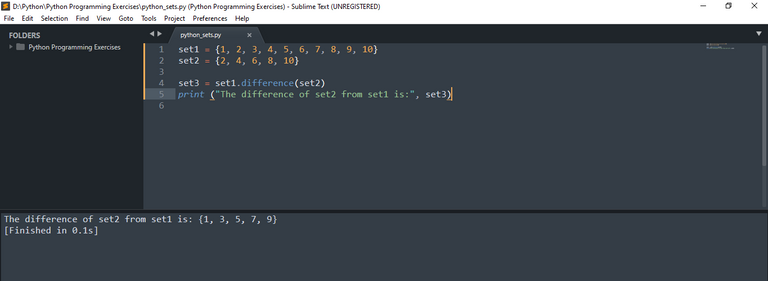This prints the element of set1 after common elements from both sets have been removed. If you want to include the element of set2 after finding difference, you can use `symmetric_difference()`. See the code below:

``````set1 = {1, 2, 3, 4, 5, 6, 7, 8, 9, 10}
set2 = {2, 4, 6, 8, 10, 12, 14}

set3 = set1.symmetric_difference(set2)
print ("The symmetric difference of set2 from set1 is:", set3)
``````

You can see in the output below that remaining element {12, 14} has also been printed along with remaining element of set1.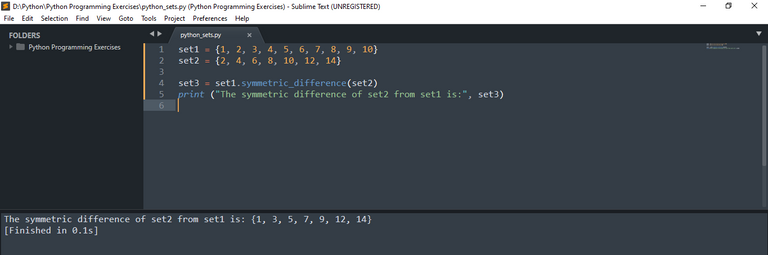You can also convert a list to a set. Just use `set()` function in order to convert any list into set. And also note that if you have duplicate elements in your list, then applying set function will remove that duplicate elements. See the following code for your reference:

``````list1 = [1, 3, 5, 7, 9, 5, 7]
print("The original list is:", list1)

set1 = set(list1)
print("\nThe corresponding set of the list1 is", set1)
``````

The output will be as below: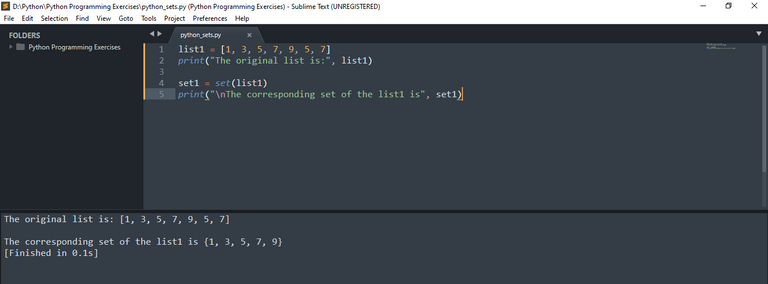There are other methods you can use in set as above. For example, you can use `clear()` method if you want to clear all the elements of your sets. Also you can try `isdisjoint()` function that will return a True value if sets have any intersection value. For example, see in below code:

``````set1 = {1, 3, 5, 6, 8, 11}
set2 = {2, 4, 10}

print(set1.isdisjoint(set2))
``````

There is no any intersecting values in these two sets. So, it should return True if you execute your code: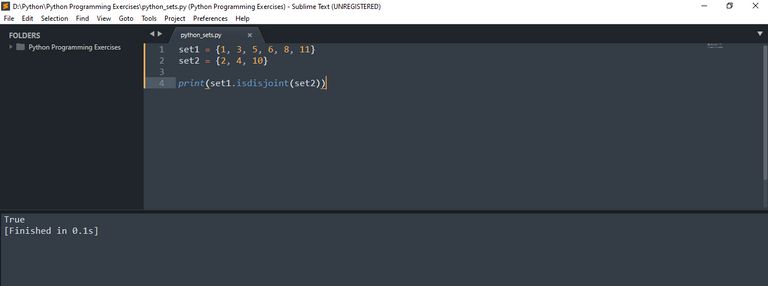Also, lets see another common set method: `issubset()` that returns True if one set is subset of another.

``````set1 = {1, 3, 5, 6, 8, 10, 11}
set2 = {6, 8, 10}

print(set2.issubset(set1))
``````

As you can see here, all elements of set2 are already in set1 so set2 is subset of s1. And if you run this code, you should see True value as below: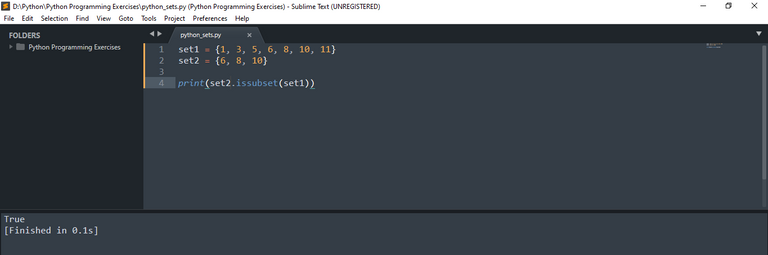Sort:

cool overview!
a nice feature of sets is that new values can also easily be added with operations like `|=`, overlap with `&`, disjoined with `^`, and more...